• 向量 a⃗=(x1,y1)，b⃗=(x2,y2)\vec a=(x_1,y_1)，\vec b=(x_2,y_2)a=(x1​,y1​)，b=(x2​,y2​)，夹角为 θ\thetaθ，内为： a⃗⋅b⃗=∣a⃗∣∣b⃗∣cos⁡θ=x1x2+y1y2 \vec a \cdot \vec b=|\vec a||\vec b|...
一、点乘（内积）
有向量 $\vec a=(x_1,y_1)，\vec b=(x_2,y_2)$，夹角为 $\theta$，内积为：
$\vec a \cdot \vec b=|\vec a||\vec b|\cos\theta=x_1x_2 + y_1y_2$
几何意义：

夹角，由 $\vec a \cdot \vec b=|\vec a||\vec b|\cos\theta$ 知，当内积  $>0$，$\theta<90^\circ$，内积  $<0$，$\theta>90^\circ$，内积  $=0$，$\theta=90^\circ$。同时也可以计算 $\theta$ 的值：$\theta=arccos\frac {\vec a \cdot \vec b}{|\vec a||\vec b|}$
投影，$|\vec a|\cos\theta=\frac {\vec a \cdot \vec b}{|\vec b|}$ 表示 $\vec a$ 在 $\vec b$ 上的投影。
对偶性：$\vec a \cdot \vec b=|\vec a|(|\vec b|\cos\theta)=|\vec b|(|\vec a|\cos\theta)$
$|\vec a|(|\vec b|\cos\theta)$ 的理解是 $\vec a$ 的长度与 $\vec b$ 在 $\vec a$ 上的投影的乘积；
$|\vec b|(|\vec a|\cos\theta)$ 的理解是 $\vec b$ 的长度与 $\vec a$ 在 $\vec b$ 上的投影的乘积；
而这两个是相等的。

二、叉乘（外积）上面的公式，就是求三阶行列式。
几何意义：

上面如果不把 $\vec i,\vec j,\vec k$ 的具体指带入公式，而是写成 $\vec a \times \vec b=m\vec i+n\vec j+l\vec k$ 的形式，向量 $(m,n,l)$ 就是一个同时垂直 $\vec a$ 和 $\vec b$ 的向量，如下图：对于二维向量，$\vec a=(x_1,y_1)，\vec b=(x_2,y_2)$，按照上面的公式得：
$\vec a \times \vec b=\begin{vmatrix} x_1 & y_1 \\ x_2 & y_2 \\ \end{vmatrix}=x_1y_2-x_2y_1$，设这个数值为 $m$。
则，$|m|=|a×b|=|a| |b|\sin\theta$ （$\theta$为 $\vec a$ 和 $\vec b$ 的夹角）
且，|m| = $\vec a$ 和 $\vec b$构成的平行四边形的面积 ，如下图：判断向量的相对位置（顺逆时针）
$\vec a$ 和 $\vec b$ 如图所示：如果让 $\vec a$ 以最小角度转到 $\vec b$ 的方向，是顺时针还是逆时针呢，从图中很容易看出，但怎么用数字判断呢？
仍然是 $m=\vec a \times \vec b=x_1y_2-x_2y_1$，
当 $m>0$，$\vec a$ 逆时针转到 $\vec b$ 的角度 $<180^\circ$，
当 $m<0$，$\vec a$ 逆时针转到 $\vec b$ 的角度 $>180^\circ$，
当 $m=0$，$\vec a$ 和 $\vec b$ 共线。
直观记忆如下图：$m>0$，$\vec b$ 在蓝色部分；
$m<0$，$\vec b$ 在红色部分；
$m=0$，$\vec b$ 在分界线上（与 $\vec a$ 共线 ）。
三、扩展（坐标系引发的顺逆指针分不清事件）
我们平时默认的坐标系是这样的：但有时候的坐标系是这样的（比如数字图像中）：可以发现，同样的 $\vec a=(2,1)$ 转到  $\vec b=(1,2)$ ，在上面的坐标系中就是逆时针，而在下面的坐标系中就是顺时针，所以为了统一说明，定义了 “正旋转” ：从 $x$ 轴旋转到 $y$ 轴的方向。
所以，上面利用向量叉乘判断向量相对位置的性质描述应该为：
当 $m>0$，$\vec a$ 正旋转到 $\vec b$ 的角度 $<180^\circ$，
当 $m<0$，$\vec a$ 正旋转到 $\vec b$ 的角度 $>180^\circ$，
当 $m=0$，$\vec a$ 和 $\vec b$ 共线。
而那张直观记忆图只在我们平时默认的坐标系中才成立。


展开全文线性代数
• 向量是由n个实数组成的一个n行1列（n*1）或...向量的点乘,也叫向量的、数量，对两个向量执行点乘运算，就是对这两个向量对应位一一相乘之后求和的操作，点乘的结果是一个标量。 点乘公式 对于向量a和向量b：

向量是由n个实数组成的一个n行1列（n*1）或一个1行n列（1*n）的有序数组；

向量的点乘,也叫向量的内积、数量积，对两个向量执行点乘运算，就是对这两个向量对应位一一相乘之后求和的操作，点乘的结果是一个标量。

点乘公式

对于向量a和向量b：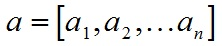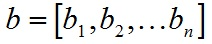a和b的点积公式为：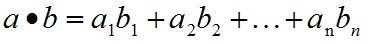要求一维向量a和向量b的行列数相同。

点乘几何意义

点乘的几何意义是可以用来表征或计算两个向量之间的夹角，以及在b向量在a向量方向上的投影，有公式：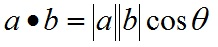推导过程如下，首先看一下向量组成：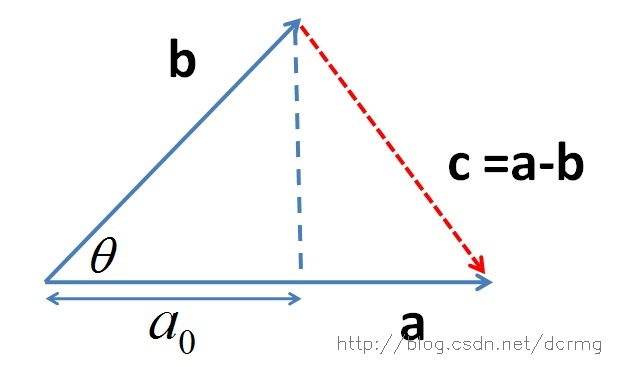定义向量：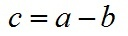根据三角形余弦定理有：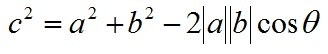根据关系c=a-b（a、b、c均为向量）有：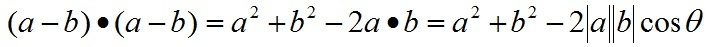即：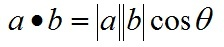向量a，b的长度都是可以计算的已知量，从而有a和b间的夹角θ：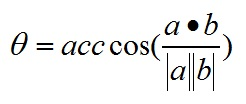根据这个公式就可以计算向量a和向量b之间的夹角。从而就可以进一步判断这两个向量是否是同一方向，是否正交(也就是垂直)等方向关系，具体对应关系为：

a·b>0    方向基本相同，夹角在0°到90°之间
a·b=0    正交，相互垂直
a·b<0    方向基本相反，夹角在90°到180°之间

叉乘公式

两个向量的叉乘，又叫向量积、外积、叉积，叉乘的运算结果是一个向量而不是一个标量。并且两个向量的叉积与这两个向量组成的坐标平面垂直。

对于向量a和向量b：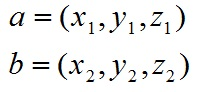a和b的叉乘公式为：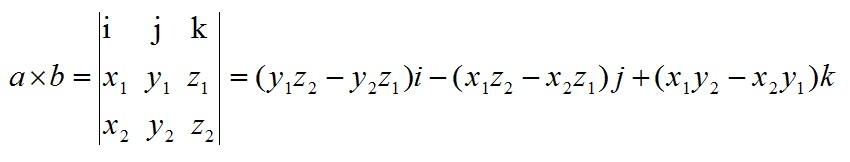其中：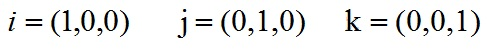根据i、j、k间关系，有：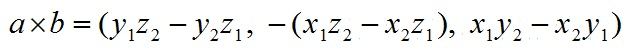叉乘几何意义

在三维几何中，向量a和向量b的叉乘结果是一个向量，更为熟知的叫法是法向量，该向量垂直于a和b向量构成的平面。

在3D图像学中，叉乘的概念非常有用，可以通过两个向量的叉乘，生成第三个垂直于a，b的法向量，从而构建X、Y、Z坐标系。如下图所示：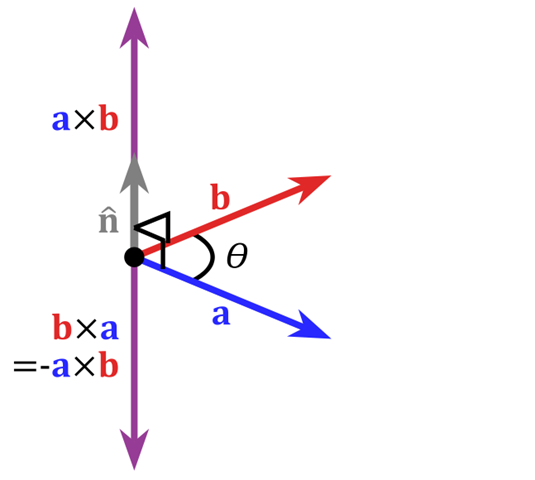在二维空间中，叉乘还有另外一个几何意义就是：aXb等于由向量a和向量b构成的平行四边形的面积。


展开全文• 参考： https://blog.csdn.net/dcrmg/article/details/52416832 ... 1 向量内积（点乘） ...a和b的点积（点乘）公式为： ...向量积的几何意义及用途 包括： 表征或计算两个向量之间的夹角 b向量在a向量...
参考：

https://blog.csdn.net/dcrmg/article/details/52416832

https://blog.csdn.net/qingzhuyuxian/article/details/84945319

1 向量内积（点乘）

公式

a和b的点积（点乘）公式为：向量内积的几何意义及用途

包括：

表征或计算两个向量之间的夹角
b向量在a向量方向上的投影积，当a是单位向量时，内积意义是投影。
当a是直线L的单位法向量时，计算b终点到L的距离

2 向量外积（叉乘）

2.1 公式：

对于向量a和向量b：a和b的外积公式为：几何意义及用途：

在三维几何中，向量a和向量b的外积结果是一个向量，有个更通俗易懂的叫法是法向量，该向量垂直于a和b向量构成的平面。

常用于以下情况：

通过两个向量的外积，生成第三个垂直于a，b的法向量，从而构建X、Y、Z坐标系；
当a是单位向量时，计算b终点到a所在直线的距离
在二维空间中，aXb等于由向量a和向量b构成的平行四边形的面积。


展开全文• 本篇内容主要是对线性代数中向量点积和外积补充,同时说明一下向量的四则运算，如果对向量和向量空间相关含义有所遗忘的话可以回顾：1、算法工程师的数学基础｜线性代数中的向量和向量空间 数学中的运算包括：加减...
线性代数主要包含向量、向量空间（或称线性空间）以及向量的线性变换和有限维的线性方程组。本篇文章主要介绍线性代数部分中的向量和向量空间。

本篇内容主要是对线性代数中向量点积和外积补充,同时说明一下向量的四则运算，如果对向量和向量空间相关含义有所遗忘的话可以回顾：1、算法工程师的数学基础｜线性代数中的向量和向量空间

数学中的运算包括：加减乘除，同样向量的运算也包括这几项，需要注意的是，保证向量的维度相同。

1、向量加减法

加法和减法是可逆的运算，比如加一个数m可以转变为减去这个数m的负数-m。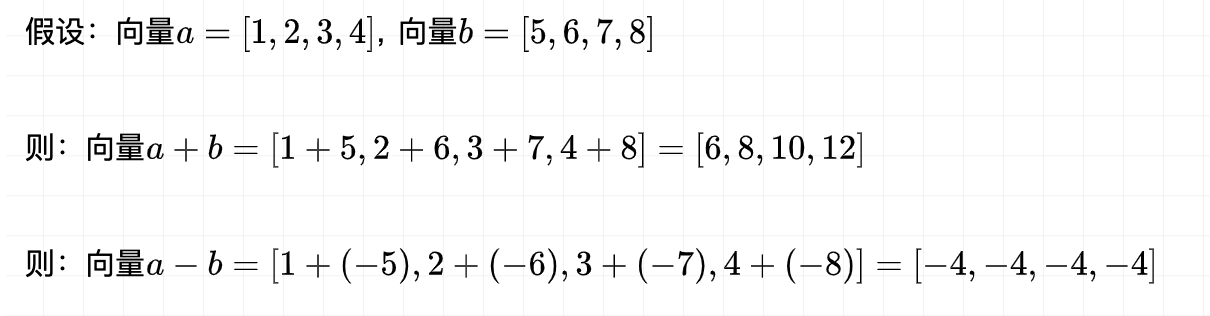2、向量乘法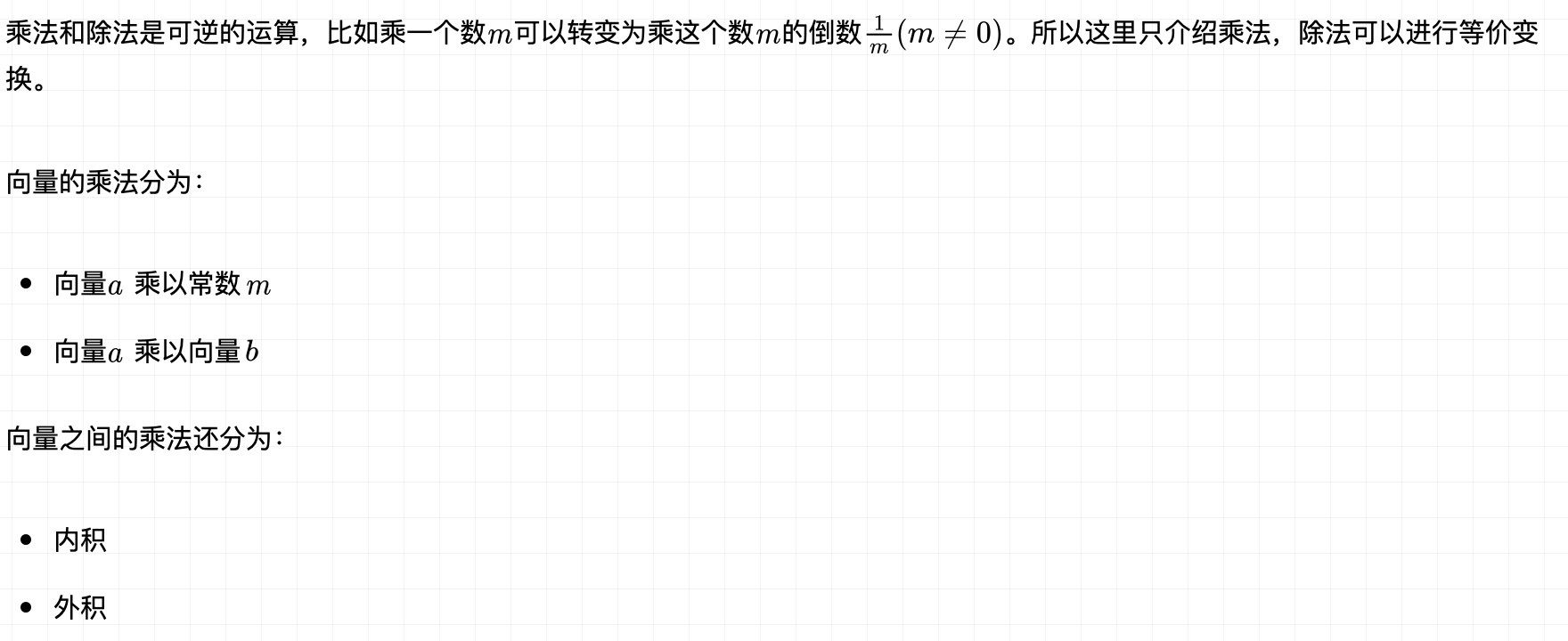3、向量乘以常数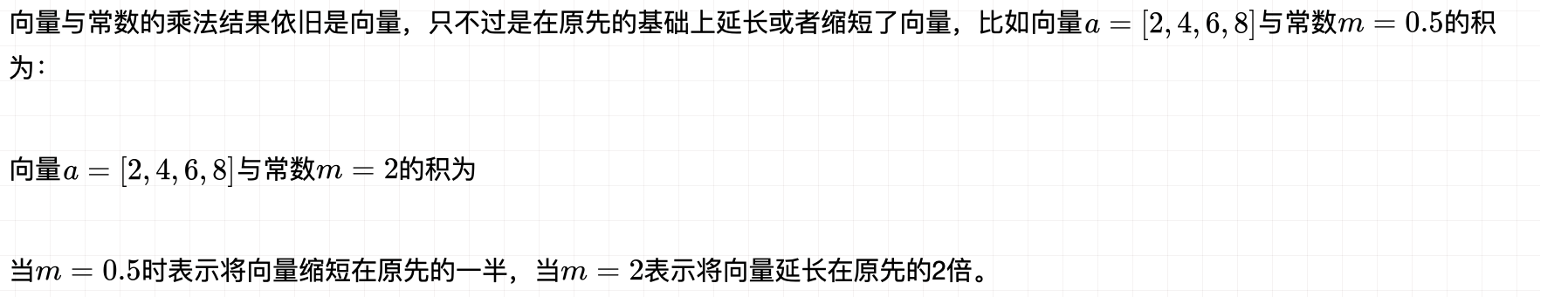4、内积

向量的内积又称为点乘或者数量乘。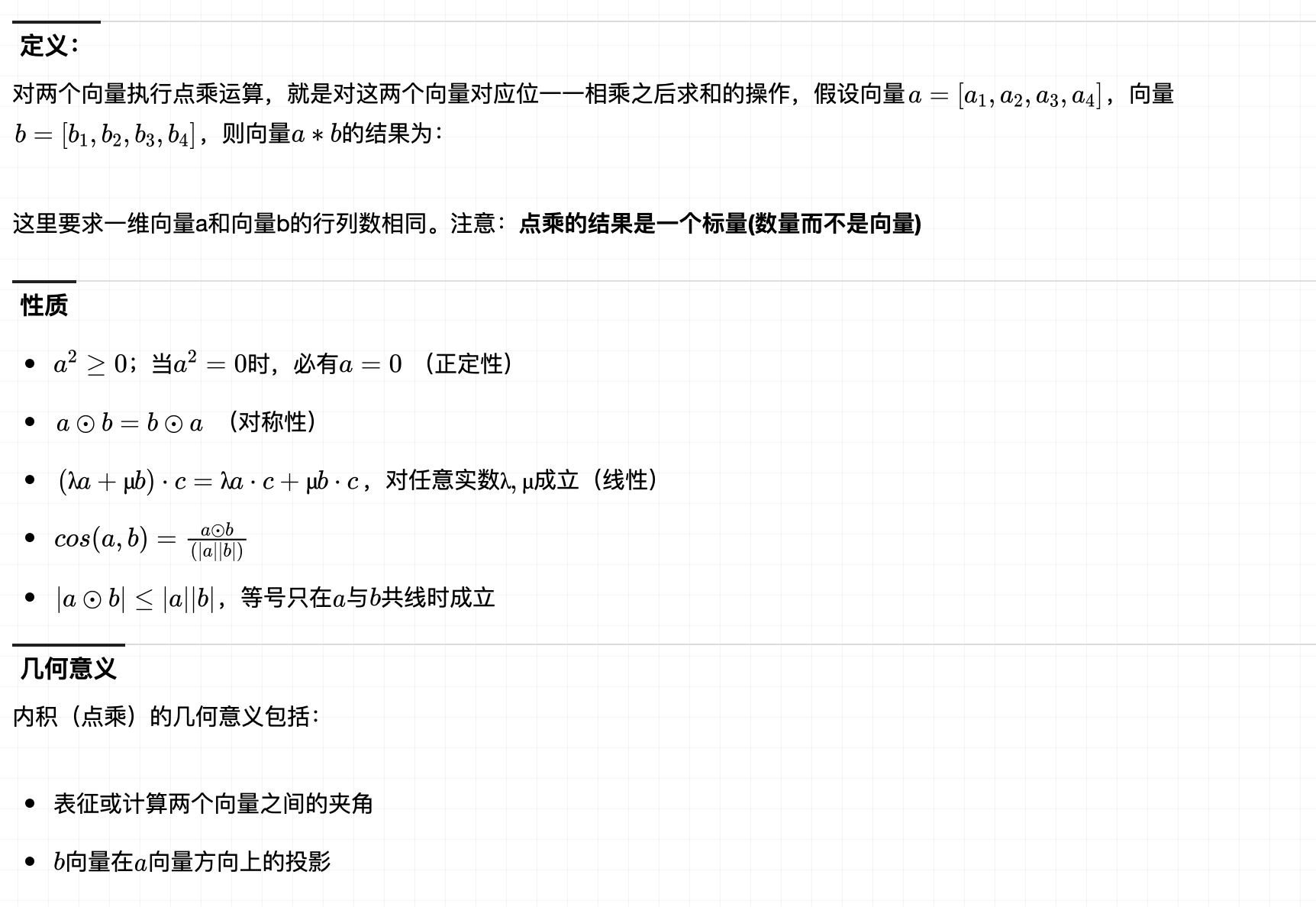5、外积

两个向量的外积又叫叉乘或者叉乘向量积，其运算结果是一个向量，并且两个向量的外积与这两个向量组成的平面垂直。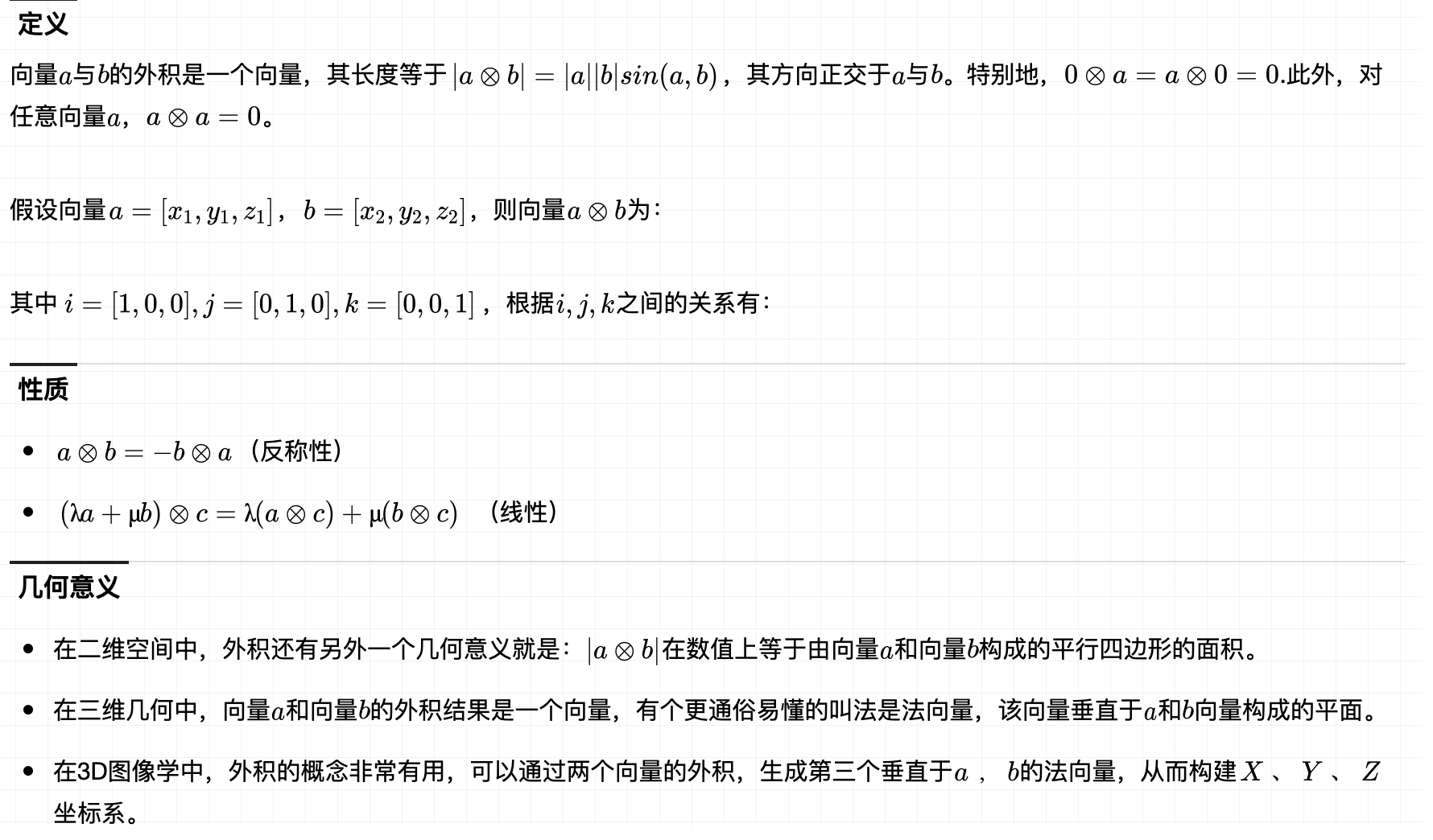展开全文• 目录1.向量2.内积3.外积4.混合积5.双重外积6.关系式正文1.向量vector 引入vector O规定O...在自由向量的意义下，相等的向量都看作是同一个向量。规定映射关系，为矢量法则。有数乘关系。2，inner product（内积） T...
• 向量是由n个实数组成的一个n行1列（n*1）或一个1行n列（1*n）的有序数组；...向量的点乘,也叫向量的、数量，对两个向量执行点乘运算，就是对这两个向量对应位一一相乘之后求和的操作，点...点乘
• 2、内积的几何意义 点乘的几何意义是可以用来表征或计算两个向量之间的夹角，以及在b向量在a向量方向上的投影。 二、向量的外积和几何意义（叉乘） 两个向量的外积，又叫向量积、叉乘等。外积的运算结果是一个...
• 向量的点乘,也叫向量的、数量，对两个向量执行点乘运算，就是对这两个向量对应位一一相乘之后求和的操作，点乘的结果是一个标量。 点乘公式 对于向量a和向量b： &nbsp; &nbsp; &nbsp; &...点乘
• 内积 计算公式 几何意义 两个向量之间夹角 向量b在向量a上投影 ...外积 ...外积在数值上等于两个向量组成平行四边形面积 reference https://www.cnblogs.com/gxcdream/p/7597865.html ...
• 向量点乘（内积）和叉乘（外积、向量积）概念及几何意义解读 向量是由n个实数组成的一个n行1列（n*1）或一个1行n列（1*n）的有序数组； 向量的点乘,也叫向量的内积、数量积，对两个向量执行点乘运算，就是对这两...
• 向量点乘(内积)和叉乘(外积、向量积)概念及几何意义解读(经典)。向量是由n个实数组成的一个n行1列(n*1)或一个1行n列(1*n)的有序数组；向量的点乘,也叫向量的内积、数量积，对两...点乘几何意义点乘的几何意义是可以...
• 2、内积的几何意义点乘的几何意义是可以用来表征或计算两个向量之间的夹角，以及在b向量在a向量方向上的投影。二、向量的外积和几何意义（叉乘）两个向量的外积，又叫向量积、叉乘等。外积的运算结果是一个向量而...
• 【转】向量点乘(内积)和叉乘(外积、向量积)概念及几何意义解读向量是由n个实数组成的一个n行1列(n*1)或一个1行n列(1*n)的有序数组；向量的点乘,也叫向量的内积、数量积，对...点乘几何意义点乘的几何意义是可以用来...
• 向量是由n个实数组成的一个n行1列(n*1)或一个1行n列(1*n)的有序数组；向量的点乘,也叫向量的、数量，对两个向量执行点乘运算，就是对这两个...点乘几何意义点乘的几何意义是可以用来表征或计算两个向量之间的......